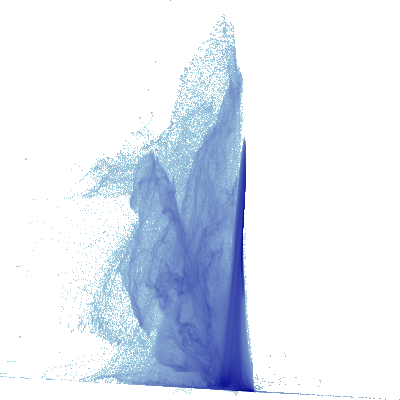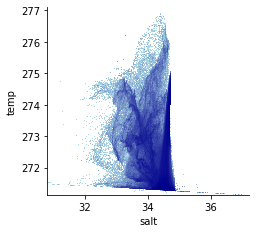# Temperature Salinity Plots¶

In this notebook there are a few examples on how to plot temperature-salinity diagrams of model output. The cosima cookbook is used to extract data, however there are other methods including using dask to get variables that could also be used, that are contained (but are outdated) in previous versions of this notebook.

The first method uses pandas dataframe to plot the data with datashader, and then the second method uses pandas dataframe to add a colourmap from the age variable. The advantage of this method is that it is efficient, but the datashader module is not as simple and user friendly as matplotlib.

The third method uses xarray scatter plots to plot a T-S diagram without pandas, instead with the xarray DataArray object. Its limitation is that it is slow.

The fourth method uses xhistogram to bin the data so that the number of points at one T-S value is clearer.

The fifth method is a documented example which uses the DataArray to make a 2D scipy histogram so that a true volume weighted T-S plot can be constructed, which takes ito account the differing sizes of cells in the model. The density isopycnals are also included in here too because they are often useful for these types of plots.

Firstly, we load our modules as required.

import dask
import numpy as np
import xarray as xr
from distributed.diagnostics.progressbar import progress
import cosima_cookbook as cc
import bokeh.plotting as bp

import matplotlib.pyplot as plt
import pandas as pd

import gsw
import scipy as scipy
from scipy import stats
from xhistogram.xarray import histogram

import holoviews as hv

from dask.distributed import Client

print('starting distributed client...')
client = Client()
display(client)

starting distributed client...


### Cluster

• Workers: 8
• Cores: 48
• Memory: 202.49 GB

The usual cosima-cookbook loading of variables…

session = cc.database.create_session('/g/data/ik11/databases/cosima_master.db')

expt = '01deg_jra55v13_ryf9091'


We load only one year of daily data and for a small portion of the ocean. If one loads the global ocean dataset the computations take very very very long.

(Have a look at the discussion in https://github.com/COSIMA/cosima-recipes/pull/75)

start_time, end_time = '2059-01-01', '2060-01-01'
temp = cc.querying.getvar(expt, 'temp', session, 'ocean.nc',
start_time=start_time, end_time=end_time).sel({'time': slice(start_time, end_time), 'xt_ocean': slice(-195, -175), 'yt_ocean': slice(-78, -68)})
salt = cc.querying.getvar(expt, 'salt', session, 'ocean.nc',
start_time=start_time, end_time=end_time).sel({'time': slice(start_time, end_time), 'xt_ocean': slice(-195, -175), 'yt_ocean': slice(-78, -68)})


## Plotting T-S diagrams with pandas and dask dataframe¶

We first construct the dataframe in pandas of the temperature and salinity data points. The dataframe matches temperature and salinity values from the input data which has been reshaped into a one dimensional array, and places them in the dataframe.

t_blocks = [x.reshape((-1, )) for x in temp.data.to_delayed().reshape((-1, ))]
s_blocks = [x.reshape((-1, )) for x in salt.data.to_delayed().reshape((-1, ))]
dfs = [delayed(pd.DataFrame)({'temp': t, 'salt': s}, copy=False) for t, s in zip(t_blocks, s_blocks)]


Using dask.dataframe to lazily read the files…

df = dd.from_delayed(dfs)


And then we use datashader to plot the T-S diagram. The colour mapping relates to how many points have that T-S coordinate, with darkblue being used to indicate a greater number of datapoints at that datapoint.

cvs = ds.Canvas(plot_width=400, plot_height=400)#, x_range =(31, 36), y_range=(-2, 3))
agg = cvs.points(df, 'salt', 'temp')
img = tf.shade(agg, cmap=['lightblue', 'darkblue'], how='log')


And now, display a T-S plot of the global ocean.

display(img)As shown above, datashader creates a very detailed image of the T-S plot in a relatively small amount of time by using dask. Datashader is also efficient for large datasets because it plots an accurate distribution of the datapoints given the size of your image, rather than plotting every datapoint as a simpler module like matplotlib does.

The above image is simply an image, but we can explort it into plotting software such as bokeh and matplotlib so that axes and labels can be added. Here we show the simplest T-S plot with datashader in each of these plotting programs, but more features could be added if you like.

hd.shade.cmap=["lightblue", "darkblue"]
hv.extension("bokeh", "matplotlib")hv.output(backend="matplotlib")
# agg = ds.Canvas().points(df, 'x', 'y')hv.output(backend="bokeh")


## Forming a pandas dataframe and plotting with a third variable¶

In the first example we simply calculated the T-S distribution and coloured it by the number of objects at that coordinate. In this example, we colour data points with a third variable, so each cell has a salinity, temperature and age parameter.

Once again we load our variables, this time for a smaller section (the Ross Sea).

start_time, end_time = '2059-01-01', '2060-01-01'

temp = cc.querying.getvar(expt, 'temp', session, 'ocean.nc',
start_time=start_time, end_time=end_time).sel({'time': slice(start_time, end_time), 'xt_ocean': slice(-195, -175), 'yt_ocean': slice(-78, -68)})
salt = cc.querying.getvar(expt, 'salt', session, 'ocean.nc',
start_time=start_time, end_time=end_time).sel({'time': slice(start_time, end_time), 'xt_ocean': slice(-195, -175), 'yt_ocean': slice(-78, -68)})
age_global = cc.querying.getvar(expt, 'age_global', session, 'ocean.nc',
start_time=start_time, end_time=end_time).sel({'time': slice(start_time, end_time), 'xt_ocean': slice(-195, -175), 'yt_ocean': slice(-78, -68)})

age_global

xarray.DataArray
'age_global'
• time: 12
• st_ocean: 75
• yt_ocean: 237
• xt_ocean: 200
• dask.array<chunksize=(1, 7, 226, 200), meta=np.ndarray>


Array  Chunk

Bytes  170.64 MB   1.27 MB
Shape  (12, 75, 237, 200)   (1, 7, 226, 200)
Type  float32  numpy.ndarray

12
1

200
237
75


• st_ocean
(st_ocean)
float64
0.5413 1.681 ... 5.709e+03
long_name :
tcell zstar depth
units :
meters
cartesian_axis :
Z
positive :
down
edges :
st_edges_ocean
array([5.412808e-01, 1.680735e+00, 2.939953e+00, 4.331521e+00, 5.869350e+00,
7.568810e+00, 9.446885e+00, 1.152234e+01, 1.381593e+01, 1.635055e+01,
1.915154e+01, 2.224687e+01, 2.566746e+01, 2.944746e+01, 3.362460e+01,
3.824057e+01, 4.334140e+01, 4.897796e+01, 5.520640e+01, 6.208874e+01,
6.969342e+01, 7.809601e+01, 8.737988e+01, 9.763700e+01, 1.089687e+02,
1.214869e+02, 1.353144e+02, 1.505868e+02, 1.674530e+02, 1.860765e+02,
2.066365e+02, 2.293296e+02, 2.543701e+02, 2.819920e+02, 3.124492e+02,
3.460166e+02, 3.829906e+02, 4.236883e+02, 4.684475e+02, 5.176242e+02,
5.715899e+02, 6.307275e+02, 6.954248e+02, 7.660668e+02, 8.430255e+02,
9.266482e+02, 1.017244e+03, 1.115068e+03, 1.220309e+03, 1.333076e+03,
1.453384e+03, 1.581154e+03, 1.716205e+03, 1.858264e+03, 2.006975e+03,
2.161913e+03, 2.322601e+03, 2.488533e+03, 2.659189e+03, 2.834054e+03,
3.012631e+03, 3.194453e+03, 3.379089e+03, 3.566145e+03, 3.755274e+03,
3.946166e+03, 4.138551e+03, 4.332197e+03, 4.526903e+03, 4.722497e+03,
4.918835e+03, 5.115794e+03, 5.313270e+03, 5.511177e+03, 5.709443e+03])
• yt_ocean
(yt_ocean)
float64
-77.98 -77.94 ... -68.06 -68.01